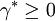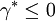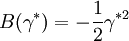Duh Henderson

(diff) ← Older revision | Latest revision (diff) | Newer revision → (diff)
The Duh-Henderson bridge function  retains the Duh Haymet bridge function for$\gamma^{*} \geq 0$, and for$\gamma^{*} \leq 0$ (Eq. 17):$B(\gamma^{*})= - \frac{1}{2} \gamma^{*2}$